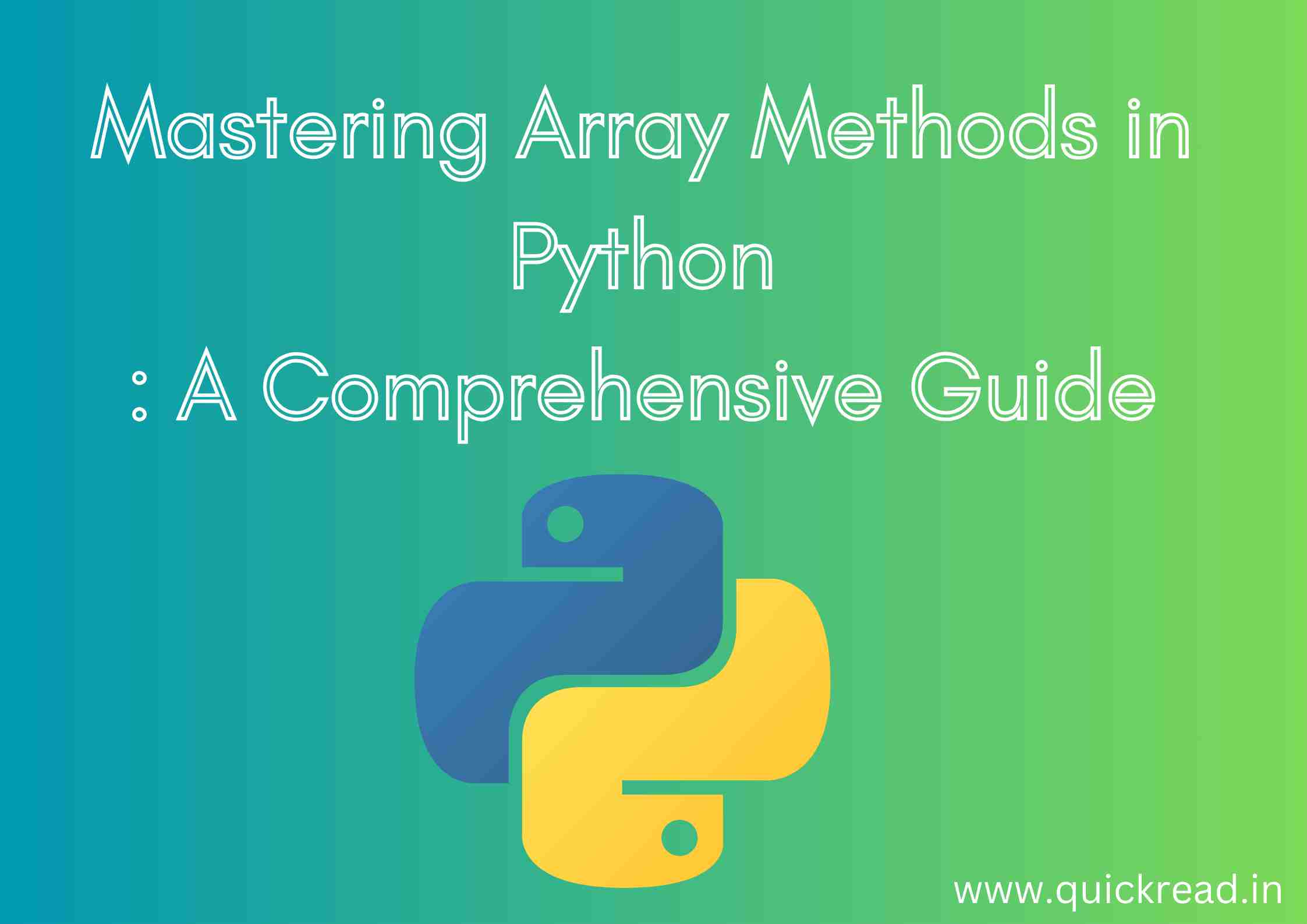# Mastering Array Methods in Python: A Comprehensive Guide## Introduction

Welcome to our comprehensive exploration of array methods in Python. Whether you’re a seasoned Python developer or someone just starting with the language, understanding array methods is essential for effective data manipulation and analysis.

Arrays serve as the backbone for storing and organizing data in Python, and Python provides a versatile toolkit of array methods to help you work with data efficiently. In this guide, we’ll take a deep dive into these array methods, shedding light on their capabilities, real-world applications, and how they can enhance your data handling skills.

## Creating Arrays

Python arrays can be declared by importing the `array` module:

``````import array

int_array = array.array('i')``````

Here we create an integer array `int_array`. The first argument specifies the data type that the array will hold. Some common ones are:

• ‘i’ – signed integer
• ‘I’ – unsigned integer
• ‘f’ – float
• ‘d’ – double

We can also initialize an array with elements:

``nums = array.array('i', [1, 2, 3])``

## Accessing Elements

Array elements can be accessed via indices like lists:

``````print(nums) # 1

nums = 5 # Update value``````

Negative indices can be used to access elements from end:

``print(nums[-1]) # 3``

`len()` function returns the array length:

``length = len(nums) # 3``

The `append()` method appends an element to the end:

``````nums.append(4)

print(nums) # [1, 5, 3, 4]``````

`insert()` can insert an element at a specific index:

``````nums.insert(1, 6)

print(nums) # [1, 6, 5, 3, 4]``````

`extend()` joins another array’s elements:

``````new_nums = array.array('i', [7, 8])
nums.extend(new_nums)

print(nums) # [1, 6, 5, 3, 4, 7, 8]``````

## Removing Elements

`remove()` removes the first occurrence of a value:

``````nums.remove(5)

print(nums) # [1, 6, 3, 4, 7, 8]``````

`pop()` removes an element at specified index:

``````nums.pop(2)

print(nums) # [1, 6, 4, 7, 8]``````

## Slicing Arrays

Just like lists, arrays can be sliced to extract sections:

``````# First 3 elements
nums[:3] # [1, 6, 4]

# 2nd to 4th element
nums[1:4] # [6, 4, 7]``````

## Finding Elements

`index()` returns the index of first occurrence of a value:

``print(nums.index(4)) # 2``

`count()` counts occurrences of a value:

``print(nums.count(7)) # 1``

`in` operator checks if a value exists:

``````if 8 in nums:
print('Exists') # Exists``````

## Sorting Arrays

The `sort()` method sorts the array in-place:

``````nums.sort()

print(nums) # [1, 4, 6, 7, 8]``````

For descending sort, set `reverse=True`:

``````nums.sort(reverse=True)

print(nums) # [8, 7, 6, 4, 1]``````

`sorted()` can be used to get a new sorted copy without modifying original.

## Reversing an Array

`reverse()` reverses the array in-place:

``````nums.reverse()

print(nums) # [1, 4, 6, 7, 8]``````

## Concatenating Arrays

Multiple arrays can be joined using `+` operator:

``````array1 = array.array('i', [1, 2, 3])
array2 = array.array('i', [4, 5, 6])

array3 = array1 + array2

print(array3) # [1, 2, 3, 4, 5, 6]``````

This creates a new array leaving the original arrays unaltered.

## Converting to List

An array can be converted to a Python list using `tolist()` method:

``````num_list = nums.tolist()
print(type(num_list)) # <class 'list'>``````

The list allows direct use of other list operations like append.

## All array methods in python in a glance

Here is a table listing Python array methods with examples

1. How are arrays different from lists in Python?

Arrays can only store numeric data types unlike lists. Arrays are more space and time efficient for large numeric data since they are restricted to primitives.

1. When should we use arrays vs lists in Python?

Use arrays when you need an efficient store for large amounts of numerical data. Use lists for non-numeric or mixed data types.

1. What are some common array operations supported in Python?

Indexing, slicing, sorting, adding/removing elements, reversing, finding elements are all supported by Python’s array module.

1. How can we concatenate two arrays in Python?

We can use the + operator to join two arrays in Python. This will create a new combined array.

1. How do you convert an array to a list in Python?

Using the tolist() method on the array will return a Python list with the same elements.

## Conclusion

Python’s array module provides a robust multi-dimensional array implementation for numeric data. Arrays allow efficient storage and access of contiguous data elements. The python array methods make it easy to manipulate arrays in an intuitive way. Arrays can serve as an alternative to lists in cases where efficient storage and array-specific routines are desirable.